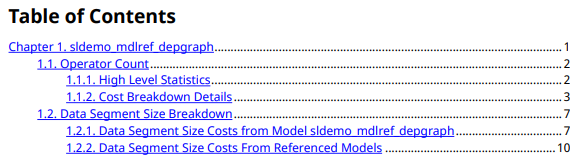# metric.Result

Metric data for specified metric algorithm

## Description

A `metric.Result` object contains the metric data for a specified metric algorithm that traces to the specified unit.

## Creation

### Syntax

``metric_result = metric.Result``

### Description

example

````metric_result = metric.Result` creates a handle to a metric result object.Alternatively, if you collect results by executing a `metric.Engine` object, using the `getMetrics` function on the engine object returns the collected `metric.Result` objects in an array.```

## Properties

expand all

Metric identifier for metric algorithm that calculates results, returned as a string.

Example: `'DataSegmentEstimate'`

Testing artifacts for which metric is calculated, returned as a structure or an array of structures. For each artifact that the metric analyzes, the returned structure contains these fields:

• `UUID` — Unique identifier of artifact

• `Name` — Name of artifact

• `Type` — Type of artifact

• `ParentUUID` — Unique identifier of file that contains artifact

• `ParentName` — Name of the file that contains artifact

• `ParentType` — Type of file that contains artifact

Result value of the metric for specified algorithm and artifacts, returned as an integer, string, double vector, or structure. For a list of metrics and their result values, see Design Cost Model Metrics and Model Testing Metrics (Simulink Check).

Scope of metric results, returned as a structure. The scope is the unit for which the metric collected results. The structure contains these fields:

• `UUID` — Unique identifier of unit

• `Name` — Name of unit

• `Type` — Type of unit

• `ParentUUID` — Unique identifier of file that contains unit

• `ParentName` — Name of file that contains unit

• `ParentType` — Type of file that contains unit

User data provided by the metric algorithm, returned as a string.

## Examples

collapse all

Use a `metric.Engine` object to collect design cost metric data on a model reference hierarchy in a project.

To open the project, enter this command.

`dashboardCCProjectStart`

The project contains `db_Controller`, which is the top-level model in a model reference hierarchy. This model reference hierarchy represents one design unit.

Create a `metric.Engine` object.

`metric_engine = metric.Engine();`

Update the trace information for `metric_engine` to reflect any pending artifact changes.

`updateArtifacts(metric_engine)`

Create an array of metric identifiers for the metrics you want to collect. For this example, create a list of all available design cost estimation metrics.

`metric_Ids = getAvailableMetricIds(me,'App','DesignCostEstimation')`
```metric_Ids = 1×2 string array "DataSegmentEstimate" "OperatorCount"```

To collect results, execute the metric engine.

`execute(metric_engine,metric_Ids);`

Because the engine was executed without the argument for `ArtifactScope`, the engine collects metrics for the `db_Controller` model reference hierarchy.

Use the `getMetrics` function to access the high-level design cost metric results.

```results_OperatorCount = getMetrics(metric_engine,'OperatorCount'); results_DataSegmentEstimate = getMetrics(metric_engine,'DataSegmentEstimate'); disp(['Unit: ', results_OperatorCount.Artifacts.Name]) disp(['Total Cost: ', num2str(results_OperatorCount.Value)]) disp(['Unit: ', results_DataSegmentEstimate.Artifacts.Name]) disp(['Data Segment Size (bytes): ', num2str(results_DataSegmentEstimate.Value)])```
```Unit: db_Controller Total Cost: 334 Unit: db_Controller Data Segment Size (bytes): 151```

The results show that for the `db_Controller` model, the estimated total cost of the design is 334 and the estimated data segment size is 151 bytes.

Use the `generateReport` function to access detailed metric results in a pdf report. Name the report `'MetricResultsReport.pdf'`.

```reportLocation = fullfile(pwd,'MetricResultsReport.pdf'); generateReport(metric_engine,'App','DesignCostEstimation','Type','pdf','Location',reportLocation);```

The report contains a detailed breakdown of the operator count and data segment estimate metric results.## Version History

Introduced in R2022a